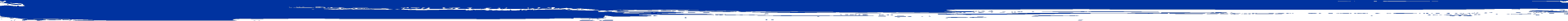# Mathematics Major Learning Goals and Outcomes

### Learning Goals

1. Students should develop effective critical thinking and communication skills within the various fields in the mathematical sciences.
2. Students will learn to use technological tools both as a tool for solving problems and as an aid to exploring ideas.
3. Students will learn to link applications to theory.
4. Students will develop mathematical independence and experience open-ended inquiry.
5. Students will be prepared for standardized tests in their chosen field.

### Learning Outcomes

1. Throughout the Mathematics major, all students will interpret, reconstruct, and critique mathematical proofs and mathematically/statistically rigorous arguments.
2. All Mathematics majors, upon completion of the major, will be able to construct clear, coherent, and logical proofs, including proofs by contradiction and induction.
3. All Mathematics majors, upon completion of the major, will be able to apply statistical techniques to a variety of problems involving data, interpret the results, communicate results to others, and critique statistical analyses.
4. All Mathematics majors will be able to use technology to create their own and critique others’ graphical and numerical representations of data and functions.
5. Students, who complete a major in mathematics, will demonstrate the ability to use a Computer Algebra System (CAS) to solve problems in Calculus and Linear Algebra.
6. Students, who complete a mathematics major, will be able to describe and state major ideas and techniques in Calculus, Linear Algebra, and Real Analysis. In addition, based on the students’ concentration, they will be able to describe and state major ideas and techniques in
• Number Theory, Geometry, and Abstract Algebra (Concentrations in Mathematical Structures and Applications and Mathematics for Teaching)
• Data Science (Concentration in Data Science)
• Data Science, Probability, and Financial Mathematics (Concentration in Actuarial Science)
1. Upon completion of the Mathematics major, all students will be able to solve real-world problems using Calculus, Linear Algebra, and Statistics. In addition, all students will be able to analyze problems and formulate appropriate mathematical and/or statistical models in a variety of areas of Mathematics and Statistics.
2. Mathematics majors who complete a concentration in Mathematics for Teaching will demonstrate an awareness of cultural and historical aspects of mathematics and an ability to relate material from their mathematics courses to high school mathematics curriculum.
3. All students majoring in Mathematics will work on a project that requires them to analyze and create mathematical and/or statistical arguments and then to produce a substantial written and/or oral report.